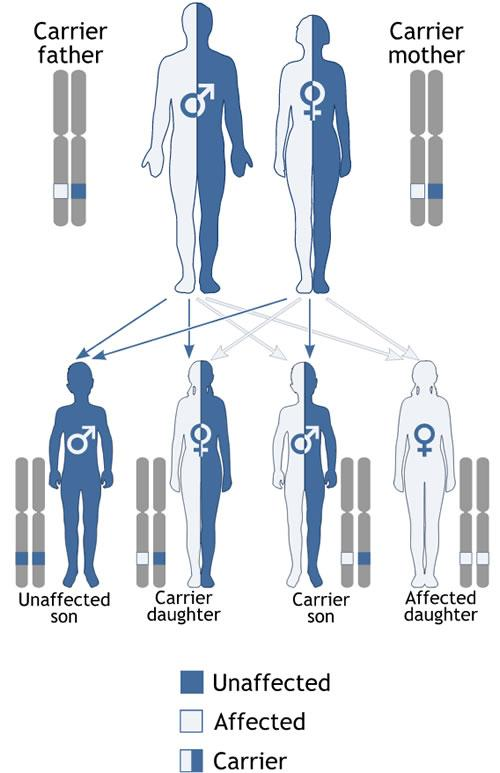### Convergence of the relative frequency to probability

#### LEGEND

Blue line:

Prediction 95% interval where we expect to find the relative frequency with the especified number of events.

Red line:

True probability. Samples are obtained ramdonly as events of that probability.

Black dashed line:

Prediction 95% interval for the relative frequency of the event at a given number of observations.

Empty dots:

Observed relative frequency at a given number of observations.

### The binomial model

The binomial model allows for computing the probability of each of the possible results of observing how many times an event will occur in a given sample. The model assumes that in each observation the probability of the event is constant. The probability of observing $$x$$ events in $$n$$ observations is computed as: $$P(X=x)= \begin{pmatrix} n\\ x \end{pmatrix} p^x (1-p)^{n-x}=\frac{n!}{x!(n-x)!}p^x (1-p)^{n-x}$$

Probability of a given result

Probability within a range

### Expected results

In the left figure, we show the probabilities of all the possible results in a sample of the indicated size. In the right figure, the possible results are shown as relative frequencies. The table shows the numeric values for the probabilities of the results. Only results with a probability higher than 0.01 are shown

## Distribution of relative frequency

Perform a simulation on the relative fequency of an event of a given probability. The red lines in the figure indicates the expected limits for the relative frequency for the 95% of the samples with n observations in each sample. You can indicate the value of probability and the number of observations per sample. Then, you can especify a number of samples and the scale of the figure. In the results panel, the percentage of samples within the theoretical limits is shown. The proportion of samples within the limits should by around a 95%. Try increasing the number of samples so that this proportion converges towards 95%.

#### Observed results

##### (c) Albert Sorribas, Ester Vilaprino, Montse Rue, Rui Alves. Biomodels Grup, Departament de Ciencies Mediques Basiques. Universitat de Lleida, Institut de Recerca Biomedica de Lleida (IRBLleida#### Probability of all the possible outcomes for a family with 4 descendants where both parents are Aa

The result with one descendant AA, one aa, and two Aa has the maximum probability, but not a high probability. In fact, it is much more probable to observe any other result.